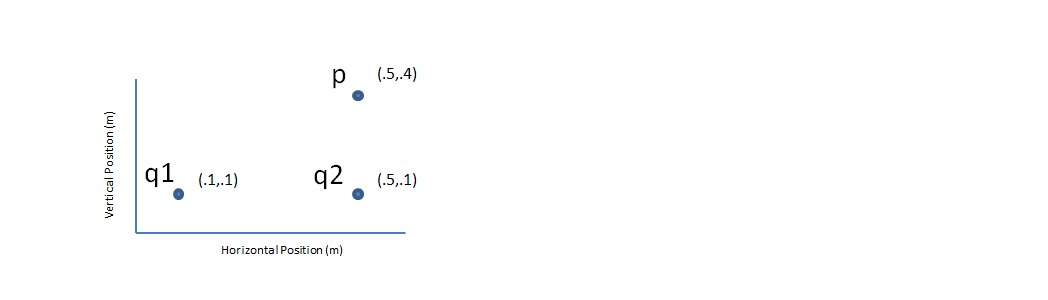# Problem: Two charges, q1 = -2.50 nC and q 2 = +2.00 nC, are placed 0.400 m apart as shown in the figure. What is the direction  of the electric field at point P, 0.300 m above q 2? A) 116° counter-clockwise from the positive x-axis. B) 63.7° clockwise from the positive x-axis. C) 72.4° counter-clockwise from the positive x-axis. D) 108° clockwise from the positive x-axis. E) 90° counter-clockwise from the positive x-axis.

###### Problem Details

Two charges, q1 = -2.50 nC and q 2 = +2.00 nC, are placed 0.400 m apart as shown in the figure. What is the direction  of the electric field at point P, 0.300 m above q 2?

A) 116° counter-clockwise from the positive x-axis.

B) 63.7° clockwise from the positive x-axis.

C) 72.4° counter-clockwise from the positive x-axis.

D) 108° clockwise from the positive x-axis.

E) 90° counter-clockwise from the positive x-axis.